# Shaft bending, making the calculation

SHAFT BENDING, MAKING THE CALCULATION S040

We all know that is a convenient method of talking about shaft deflection and this number has proven to be an accurate method of predicting premature seal and bearing failure in a single stage centrifugal pump with an overhung impeller. In the section titled shaft bending, learning the L3/D4formula I gave you the formula we use to calculate the force on the end of the shaft of a single stage centrifugal pump with an overhung impeller.

This is the most popular pump being used in the process industry today. Here again is the formula we use to calculate the hydraulic force on the end of the pump shaft: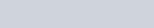• P = The resultant force, in pounds
• K = The radial thrust factor. This number comes from a chart that relates to specific speed.
• H = Total head at Q gpm measured in feet.
• D2 = Outside diameter of the impeller, measured in inches.
• B2 = Width of the impeller in inches.
• Sg. = The specific gravity of the fluid
• 2.31= The conversion from feet of head to pounds/ square inch
• Kq = A capacity factor equal to: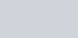• Q = The capacity in gpm at which the radial thrust is to be calculated.
• Q= The capacity in gpm at the best efficiency point (BEP) of the pump

I will be working the numbers in both the imperial and metric systems. First we will work the numbers in the imperial system and at the end of this article we will make the same calculations in the metric system.

I will use a direct conversion to metric to show you that the conversion works. In reality we would not be using these exact numbers, but it is important to develop confidence in your ability to work in either system. Because I am working with a direct conversion I will continue to use 1750 rpm or the numbers will come out differently. I am well aware that your calculations will probably be at 1450 or 2900 rpm.

We are now going to use this formula to make an actual calculation of the shaft deflection on a typical ANSI standard pump running at shut off. This is a normal starting method for centrifugal pumps of this type. The following information would have been read off the pump curve that came with the pump and a radial thrust factor chart (K) you will find if you see “shaft bending, learning the L3/D4 formula” in another part of this CD.

• P = The resultant force, in pounds
• K = 0.37 from the chart
• H = 184 feet.
• D2 = 13 inches.
• B2 = 1 inch.
• Q = 0 gpm at shut off.
• Qn = 300 gpm
• Speed = 1750 rpm.
• specific gravity 1.0

Putting these numbers into the formula we get: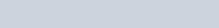If we add the weight of the impeller estimated to be ten pounds, the total force on the end of the shaft becomes 393 pounds.

Now that we have the total force, we will use this information to calculate how much the overhung shaft will bend. To make the calculation we will use the following bending formula: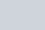“I” describes the moment of inertia for a solid, round shaft and the formula looks like this: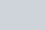Substituting this term into the bending formula we get: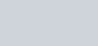• Y = The amount of shaft bending in inches.
• F = The total force on the shaft.
• L = The length of the shaft from the center of the radial bearing to the center of the impeller.
• E = The modulus of elasticity. The numbers for common shaft materials will vary from 28 to 30 million psi. (28 – 30 X106)
• D = The diameter of the solid shaft under the sleeve, if there is sleeve on the shaft.
• I = The moment of inertia for a solid round shaft: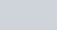If we simplify the formula we would get: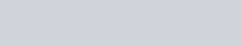Now let’s put in the actual numbers and see how much the shaft will bend with 393 pounds of force on the end of it:

• F = 393 pounds
• L = 9 inches
• D = I.5 inches with a 1.750 sleeve.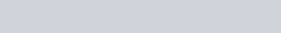Thirteen thousands of an inch bending is enough bending to cause problems with the impeller, wear rings, mechanical seals and bearings.

• The impeller could hit the pump volute or the back plate.
• The stationary and rotating wear ring components could come into contact.
• The shaft could contact the end of the stuffing box.
• The rotating part of the mechanical seal could hit the inside of the stuffing box.
• The rotating shaft could contact the inside diameter of the stationary seal face.
• The bearings could become overloaded.
• The deflection could cause excessive movement of both stationary and rotating seal designs.
• Any shaft fretting will be accelerated.

Here is the metric force formula;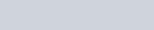Here are the original numbers converted to a metric value:

• P = The resultant force, in kilograms
• K = 0.37. from the chart
• H = 56.08 meters.
• D2 = 33.02 centimeters.
• B2 = 2.54 centimeters
• Q = 0 m3/hr. at shut off.
• Qn = 68 m3/hr.
• Speed = 1750 rpm.
• Specific gravity =1.0

Putting these numbers into the formula we get: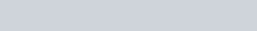If we add the weight of the impeller estimated to be 4.54 kg, the total force on the end of the shaft becomes 182.12 kg.

Now that we have the total force, we will use this information to calculate how much the overhung shaft will bend. To make the calculation we will use the following bending formula:“I” describes the moment of inertia for a solid, round shaft and the formula looks like this:Substituting this term into the bending formula we get:• Y = The amount of shaft bending in centimeters
• F = The total force on the shaft in kilograms.
• L = The length of the shaft from the center of the radial bearing to the center of the impeller in centimeters.
• E = The modulus of elasticity. The numbers for common shaft materials will vary from 1.96 to 2.1 million kilograms per square centimeter)
• D = The diameter of the solid shaft under the sleeve, if there is a sleeve on the shaft, measured in centimeters.
• I = The moment of inertia for a solid round shaft: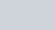Now let’s put in the actual numbers and see how much the shaft will bend with 182.12 kilograms force on the end of it:

• F = 182.12 kilograms
• L = 22.86 centimeters
• D = 3.75 centimeters.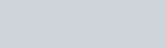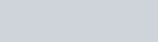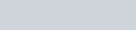A deflection of 0.036 cm. is enough to do wear ring damage to the closed impeller design commonly used in European pumps and pumps specified by petroleum refineries throughout the world.

### Posted

• On February 17, 2018